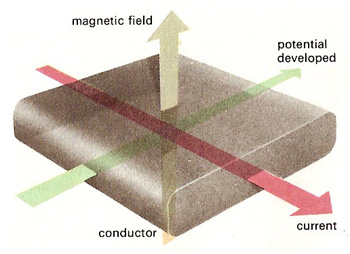A# Hall effectThe Hall effect shown schematically.

The Hall effect is the generation of an electromotive force, or a potential difference, across a conductor or semiconductor when it is carrying a current perpendicular to a magnetic field around it. The voltage is at right-angles (90°) to both the direction of the current and the direction of the magnetic field, and arises from the deflection of moving charge carriers – electrons or holes – by the magnetic field. The effect was discovered in 1879 by the American physicist Edwin H. Hall (1855–1938).

If a current (I) is passed through a conducting crystal in a direction perpendicular to that of an applied magnetic field (H), the conductor develops a potential (V) between the faces which are mutually perpendicular to both the direction of current and the magnetic field. The magnitude of the potential difference is given by

V = IH/Ned

where N is the number of current carriers, e is the electronic charge, and d is the distance of separation of the two faces across which the potential is developed.

The Hall effect can be used to test the electrical properties of materials and in ascertaining the strength of magnetic fields. It also forms the basis of the Hall effect thruster.